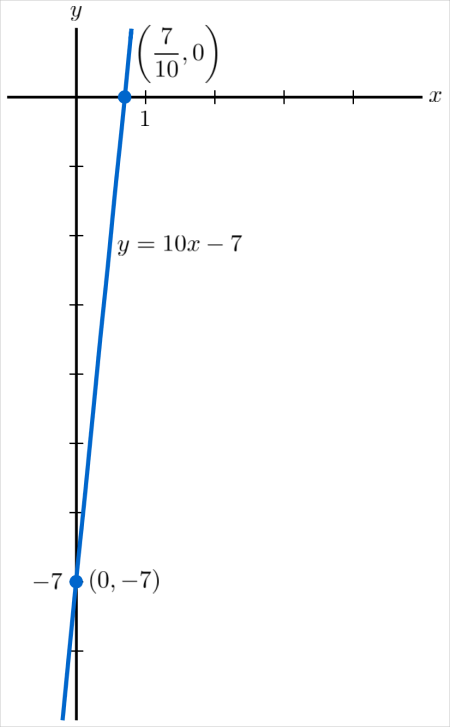# Determine whether a) (2,13) and b) (-1,-3) lie on the graph of y = 10x - 7 . c) Sketch the...

## Question:

Determine whether

a) (2,13) and

b) (-1,-3)

lie on the graph of

{eq}y = 10x - 7 {/eq}.

c) Sketch the graph by finding {eq}x- {/eq} and {eq}y- {/eq}intercepts.

## Lines and Points on the Cartesian Plane:

From geometry, two points uniquely determine a line. This means that if we have two points, it is equivalent to knowing the line that contains them. This is why we can draw the graph of an equation for a line just by determining two of the points on it. We can determine if a point is contained on a line using the equation for the line: if the coordinates satisfy the equation, that is, if the resulting equation is true when we substitute their values, then the line contains the point.

To determine if a point is on the graph of any curve, we can check and see if the coordinates of the point satisfy the given equation of the curve. To do this, we substitute the values into their respective variables and see if we end up on a true statement. If this happens, the point satisfies the equation and therefore lies on the curve.

(a) For {eq}(2, 13) {/eq}, we sub in {eq}x = 2 {/eq} and {eq}y = 13 {/eq} to the equation and get \begin{align*} y &= 10x - 7 \\ 13 &= 10(2) - 7 \\ 13 &= 20 - 7 \\ 13 &= 13 \end{align*} and since this is true, the point {eq}(2, 13) {/eq} is on the line.

(b) We do the same for the point {eq}(-1, -3) {/eq}. We have \begin{align*} y &= 10x - 7 \\ -3 &= 10(-1) - 7 \\ -3 &= -10 - 7 \\ -3 &= -17 \end{align*} and since this is false, the point does not satisfy the equation and so the point does not lie on the line.

(c) To find the intercepts of the line, we set each of {eq}x {/eq} and {eq}y {/eq} to zero for the {eq}y {/eq}- and {eq}x {/eq}-intercepts, respectively. We have \begin{align*} x = 0: y &= 10(0) - 7 \\ y &= 3 \\ y = 0: 0 &= 10x - 7 \\ 10x &= 7 \\ x &= \frac{7}{10} \end{align*} so the intercepts are at {eq}(0, -7) {/eq} and {eq}\left( \frac{7}{10}, 0 \right) {/eq}. To sketch the graph of the line, we just have to plot these two points and draw the line connecting them: